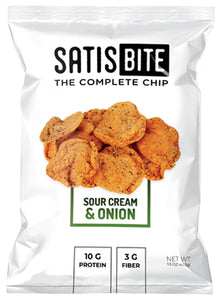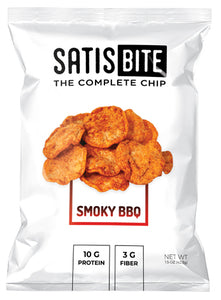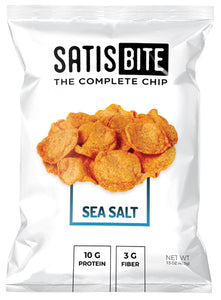FREE 2-3 DAY SHIPPING on all orders!

# Collection: Shop

4 products
• Sour Cream & OnionRegular price
Sold out
Sale price
25.99
Sold out

Box of 12

Regular price
Sold out
Sale price
25.99
Sold out
 Subtotal = 51.98 5% Discount = (2.58) Total = -2.58
 Subtotal = 623.76 Free Month = (77.97) 10% Discount = (5.18) Total = -5.18
• Smoky BBQRegular price
Sold out
Sale price
25.99
Sold out

Box of 12

Regular price
Sold out
Sale price
25.99
Sold out
 Subtotal = 51.98 5% Discount = (2.58) Total = -2.58
 Subtotal = 623.76 Free Month = (77.97) 10% Discount = (5.18) Total = -5.18
• Sea SaltRegular price
Sold out
Sale price
25.99
Sold out

Box of 12

Regular price
Sold out
Sale price
25.99
Sold out
 Subtotal = 51.98 5% Discount = (2.58) Total = -2.58
 Subtotal = 623.76 Free Month = (77.97) 10% Discount = (5.18) Total = -5.18
• Free Sample PackRegular price
Sold out
Sale price
0.00
Sold out

Box of 3

Regular price
Sold out
Sale price
0.00
Sold out
 Subtotal = 0.00 5% Discount = (0.00) Total = 0.0
 Subtotal = 0.00 Free Month = (77.97) 10% Discount = (0.00) Total = 0.0